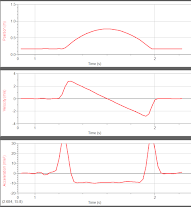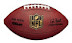Use Google's Chrome browser for proper viewing.

IB Physics SL (2019-2020)

8/15

• Informational Packet / Syllabus
• Introduction to class
• Homework - brainstorm a notation for keeping track of how far an object moves AND in which direction it travels, e.g. a man walks 20 meters eastward, then 20 meters northward, then 10 meters westward; can you write this in a way that the sum of these three motions gives you the man's final position?

8/19

• discuss briefly the history of modeling motion
• differentiate between average and instantaneous speeds and define constant speed
• determine if a toy car moves with constant speed
• create a scatter plot of the motion of a toy car and discuss the significance of its slope
• Homework - read to p. 11 in the booklet (Physics: An Introduction); do the 6 math questions on p. 10

8/21

• define distance, displacement, speed, velocity and acceleration
• "read" position, velocity and acceleration graphs and relate them to one another

8/23

8/27

• derive the kinematic (or suvat) equations
• practice using the kinematic equations
• Homework - solve sample IB test questions on motion graphs; if you have a textbook, read over pp. 27-38 for a review of the material we've covered thus far

8/29

• test over motion graphs, with general questions about distance, displacement, speed (average and instantaneous), velocity and acceleration
• Homework - install Logger Pro on your laptop (on a school-issued laptop, install it from the Software Center; on all other laptops, use these instructions); read pp. 27-38 in your textbook

9/3

• graph the motion data in this spreadsheet and add trendlines
• practice interpreting trendlines and using the extracted (velocity and acceleration) information to interpolate and extrapolate (file 1file 2)
• Extra Practice  - match motion to graphs with this interactive.
• Homework - do test corrections on a separate sheet of paper (just rework the missed problems)

9/5

• picture day
• read what Aristotle and Galileo had to say about falling "bodies"
• study how objects fall using a "motion detector" - lab instructions
• homework - finish the lab at home; read (and take notes on) pp. 8-16 in your textbook

9/9

• use the kinematic equations for modeling freely falling objects - worksheet
• homework - read about experimental uncertainty (its measurement and propagation in calculations) - handout

9/11

• begin formal lab investigation of free fall acceleration
• the general Lab Report Format

9/13

• calculate lab uncertainties
• the lab report will be due Wed 9/18 (at 11:59 pm) through turnitin.com
• homework - you should get started on the lab report

9/17

• graph the free fall data -- linearize the graph
• homework - complete your lab report; the free fall calculations are due 9/23

9/19

• quiz over free fall  postponed until Monday
• review free fall calculations
• discuss relative motion
• homework - complete free fall calculations (handout)

9/23

• quiz over free fall
• introduction to vectors
• homework - read pp. 39-43 in your textbook

9/25

• model 2D projectiles
• homework - complete this worksheet of projectile problems (the answers are given within the document)

9/27

• review projectile problem-solving
• projectile motion lab (online)
• homework - complete the lab
• pay for the IB Physics field trip to iFLY! The trip will be Mon, Oct 21.

10/1

• Mythbusters video
• discuss the effect of air resistance on projectile motion
• solve IB projectile problems
• help with projectiles
• homework - extra projectile practice (key)

10/3

• quiz over projectile motion
• discuss the nature of a "law of nature" and the certainty of our knowledge
• homework - pay for the iFLY field trip!

10/7

10/9

• meeting with counselor
• discuss the concept of a force and the types of forces found within our world (follow this guide and answer questions in your class notebook)

10/11

• review types of forces
• begin lab investigation of Newton's 2nd Law - formal lab info

**No school on Monday or Tuesday**

10/17

• complete the lab
• STUDENTS: before going to iFLY, you or your parent must complete this
• online waiver!! go ahead and do this asap -- reservation #4115004005
• homework - the formal lab write up is due...

10/21

• field trip to iFLY !!
Lab Report Format

Unit One-Page Overviews

1.1 Measurements in physics

1.2 Uncertainties and errors

1.3 Vectors and scalars

2.1 Motion

2.2 Forces

2.3 Work, Energy, and Power

2.4 Momentum

Unit One-Page Overviews

3.1 Thermal Concepts

3.2 Modelling a gas

6.1 Circular Motion

4.1 Oscillations

4.2 Travelling Waves

4.3 Wave Characteristics

5.1 Electric FieldsFree Fall Motion: s,v,&a graphs

Parabolas in the NFL: the physics of a football punt